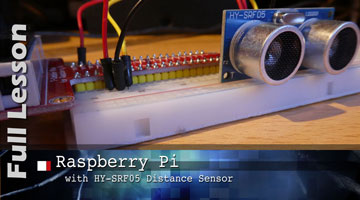# Raspberry Pi, Ultrasonic Distance Sensor HY-SRF05 - Full Lesson

### Introduction

This lesson uses a Raspberry Pi 4, an HYSRF05 distance sensor and Python to measure distance. The video has all the details.

The accompanying video does he following:

• shows how to wire the sensor to the Raspberry Pi,
• explains how the sensor works (at a high level)
• explains edges and rising and falling edges on pins
• explains using a list as a stack to store data
• explains line by line all the code necessary to get measurements from the sensor and reported through a python program

### Lesson Objectives

There are 5 things you’ll learn in this lesson:

1. Understand the difference between using polling and interrupts to monitor a sensor
2. Learn how to program a motion sensor using interrupts
3. Define rising and falling edges
4. Understand how to use a stack for temporary data storage
5. Understand the concept of threads using a callback function

### Results

By the time I worked through learning about edge detection and ironing the bugs out of my code, the final program seems fairly robust. It still occasionally generates some results that are very inaccurate but under normal conditions, when the distance isn't changing too quickly, the measurements are quite reliable.

### Source Code

import RPi.GPIO as GPIO
import time
import statistics

#### Define program constants
trigger_pin=4    # the GPIO pin that is set to high to send an ultrasonic wave out. (output)
echo_pin=17      # the GPIO pin that indicates a returning ultrasonic wave when it is set to high (input)
number_of_samples=5 # this is the number of times the sensor tests the distance and then picks the middle value to return
sample_sleep = .01  # amount of time in seconds that the system sleeps before sending another sample request to the sensor. You can try this at .05 if your measurements aren't good, or try it at 005 if you want faster sampling.
calibration1 = 30   # the distance the sensor was calibrated at
calibration2 = 1750 # the median value reported back from the sensor at 30 cm
time_out = .05 # measured in seconds in case the program gets stuck in a loop

#### Set up GPIO
GPIO.setwarnings(False)
GPIO.setmode(GPIO.BCM)

# Set up the pins for output and input
GPIO.setup(trigger_pin, GPIO.OUT)
GPIO.setup(echo_pin, GPIO.IN, pull_up_down=GPIO.PUD_DOWN)

#### initialize variables
samples_list = [] #type: list # list of data collected from sensor which are averaged for each measurement
stack = []

def timer_call(channel) :
# call back function when the rising edge is detected on the echo pin
now = time.monotonic()  # gets the current time with a lot of decimal places
stack.append(now) # stores the start and end times for the distance measurement in a LIFO stack

def trigger():
# set our trigger high, triggering a pulse to be sent - a 1/100,000 of a second pulse or 10 microseconds
GPIO.output(trigger_pin, GPIO.HIGH)
time.sleep(0.00001)
GPIO.output(trigger_pin, GPIO.LOW)

def check_distance():
# generates an ultrasonic pulse and uses the times that are recorded on the stack to calculate the distance
# Empty the samples list
samples_list.clear()

while len(samples_list) < number_of_samples:       # Checks if the samples_list contains the required number_of_samples
# Tell the sensor to send out an ultrasonic pulse.
trigger()

# check the length of stack to see if it contains a start and end time . Wait until 2 items in the list
while len(stack) < 2:                          # waiting for the stack to fill with a start and end time
start = time.monotonic()                   # get the time that we enter this loop to track for timeout
while time.monotonic() < start + time_out: # check the timeout condition
pass

trigger()                                  # The system timed out waiting for the echo to come back. Send a new pulse.

if len(stack) == 2:                          # Stack has two elements on it.
# once the stack has two elements in it, store the difference in the samples_list
samples_list.append(stack.pop()-stack.pop())

elif len(stack) > 2:
# somehow we got three items on the stack, so clear the stack
stack.clear()

time.sleep(sample_sleep)          # Pause to make sure we don't overload the sensor with requests and allow the noise to die down

# returns the media distance calculation
return (statistics.median(samples_list)*1000000*calibration1/calibration2)

###########################
# Main Program
###########################

GPIO.add_event_detect(echo_pin, GPIO.BOTH, callback=timer_call)  # add rising and falling edge detection on echo_pin (input)

for i in range(1000): # check the distance 100 times
print(round(check_distance(), 1)) # print out the distance rounded to one decimal place

See Raspberry Pi, Ultrasonic Distance Sensor HR-SRF05 - Background for more information